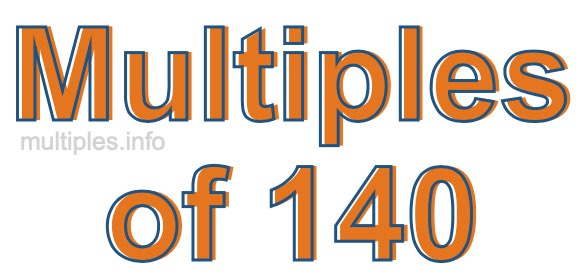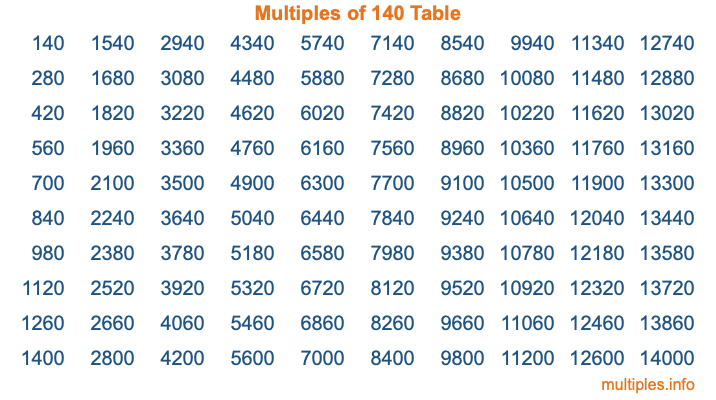Multiples of 140Welcome to the Multiples of 140 page. Here we will first teach you everything you will ever need to know about the multiples of 140, and then give you a study guide summary of everything we taught you to make sure you remember it all. Use this page to look up facts and learn information about the multiples of 140. This page will make you a multiples of one hundred forty expert!

Definition of Multiples of 140
Multiples of 140 are all the numbers that when divided by 140 equal an integer. Each of the multiples of 140 are called a multiple. A multiple of 140 is created by multiplying 140 by an integer.

Therefore, to create a list of multiples of 140, you start with 1 multiplied by 140, then 2 multiplied by 140, then 3 multiplied by 140, and so on for as long as you want. Thus, the list of the first five multiples of 140 is 140, 280, 420, 560, and 700. To see a larger list of multiples of 140, see the printable image of Multiples of 140 further down on this page. We also have a category where you can choose any nth multiple of 140.

Multiples of 140 Checker
The Multiples of 140 Checker below checks to see if any number of your choice is a multiple of 140. In other words, it checks to see if there is any number (integer) that when multiplied by 140 will equal your number. To do that, we divide your number by 140. If the the quotient is an integer, then your number is a multiple of 140.

Is  a multiple of 140?

Least Common Multiple of 140 and ...
A Least Common Multiple (LCM) is the lowest multiple that two or more numbers have in common. This is also called the smallest common multiple or lowest common multiple and is useful to know when you are adding our subtracting fractions. Enter one or more numbers below (140 is already entered) to find the LCM.

Check out our LCM Calculator if you need more details about the Least Common Multiple or if you need the LCM for different numbers for adding and subtraction fractions.

nth Multiple of 140
As we stated above, 140 is the first multiple of 140, 280 is the second multiple of 140, 420 is the third multiple of 140, and so on. Enter a number below to find the nth multiple of 140.

th multiple of 140

Multiples of 140 vs Factors of 140
140 is a multiple of 140 and a factor of 140, but that is where the similarities end. All postive multiples of 140 are 140 or greater than 140. All positive factors of 140 are 140 or less than 140.

Below is the beginning list of multiples of 140 and the factors of 140 so you can compare:

Multiples of 140: 140, 280, 420, 560, 700, etc.

Factors of 140: 1, 2, 4, 5, 7, 10, 14, 20, 28, 35, 70, 140

As you can see, the multiples of 140 are all the numbers that you can divide by 140 to get a whole number. The factors of 140, on the other hand, are all the whole numbers that you can multiply by another whole number to get 140.

It's also interesting to note that if a number (x) is a factor of 140, then 140 will also be a multiple of that number (x).

Multiples of 140 vs Divisors of 140
The divisors of 140 are all the integers that 140 can be divided by evenly. Below is a list of the divisors of 140.

Divisors of 140: 1, 2, 4, 5, 7, 10, 14, 20, 28, 35, 70, 140

The interesting thing to note here is that if you take any multiple of 140 and divide it by a divisor of 140, you will see that the quotient is an integer.

Multiples of 140 Table
Below is an image of the first 100 multiples of 140 in a table. The table is in chronological order, column by column. The first column has the first ten multiples of 140, the second column has the next ten multiples of 140, and so on.The Multiples of 140 Table is also referred to as the 140 Times Table or Times Table of 140. You are welcome to print out our table for your studies.

Negative Multiples of 140
Although not often discussed or needed in math, it is worth mentioning that you can make a list of negative multiples of 140 by multiplying 140 by -1, then by -2, then by -3, and so on, to get the following list of negative multiples of 140:

-140, -280, -420, -560, -700, etc.

Multiples of 140 Summary
Below is a summary of important Multiples of 140 facts that we have discussed on this page. To retain the knowledge on this page, we recommend that you read through the summary and explain to yourself or a study partner why they hold true.

There are an infinite number of multiples of 140.

A multiple of 140 divided by 140 will equal a whole number.

140 divided by a factor of 140 equals a divisor of 140.

The nth multiple of 140 is n times 140.

The largest factor of 140 is equal to the first positive multiple of 140.

140 is a multiple of every factor of 140.

140 is a multiple of 140.

A multiple of 140 divided by a divisor of 140 equals an integer.

140 divided by a divisor of 140 equals a factor of 140.

Any integer times 140 will equal a multiple of 140.

Multiples of a Number
Here you can get the multiples of another number, all with the same attention to detail as we did for multiples of 140 on this page.

Multiples of
Multiples of 141
Did you find our page about multiples of one hundred forty educational? Do you want more knowledge? Check out the multiples of the next number on our list!